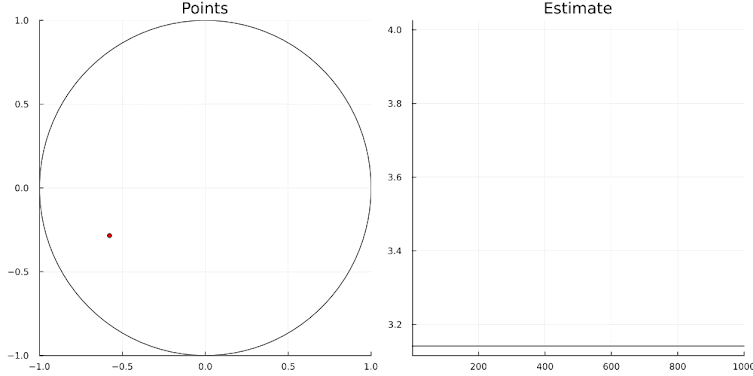## Wednesday, September 27, 2023

### Math and ChatGPT

Performing symbolic math steps is often related to pattern recognition. In theory, ChatGPT could be doing a good job here. I wanted to find the inverse of $f(x) = {\mathrm{sign}}(x) \log(1+|x|)$ This function is a form of a signed logarithmic scaling. So, let's see what ChatGPT is telling us:

## Wednesday, September 20, 2023

### Julia vs Python

I gave a talk to economists (i.e., not professional programmers) about a Julia project we were working on. Julia is famous for its speed. It uses LLVM  as back-end for its JIT (Just In Time) compilation. As seeing is believing, here is an example algorithm which is used to compare performance between different languages and tools. This example was chosen as it is small, easy to explain, and easy to program while still showing meaningful time differences.

We have a square $$[-1,+1]\times[-1,+1]$$ and an inscribing circle with radius $$1$$. See the dynamic figure below. Their areas are $$4$$ and $$\pi$$ respectively. The idea is to draw $$n$$ points \begin{align}& x_i \sim U(-1,+1) \\ & y_i \sim U(-1,+1)\end{align}Let $$m$$ be the number of points inside the circle, i.e. with $x_i^2+y_i^2\lt 1$ Obviously, from the ratio of the areas, we have $\frac{m}{n} \approx \frac{\pi}{4}$ It follows that an estimate of $$\pi$$ is $\hat{\pi}=4\frac{m}{n}$Simulation with n=1000

## Monday, September 4, 2023

### Critiquing a GAMS Model

It is always interesting to read GAMS models written by someone else. There are probably three things one can observe:

• A nice formulation or concept that is useful to learn about.
• A bad implementation: something that really should not be done that way.
• A piece of code that is correct and defensible, but I would write it differently. This includes things like style, layout, formatting, etc.
My way of reading GAMS code is often to start editing and make it "my code". It is a bit slower process, but that comes with its advantages: better understanding of what is going on, and often cleaner code.

Here, I am looking at the model sambal.gms in the GAMS model library . It is a very small model, but I have many thoughts about it. The complete model is reproduced in Appendix 1. Let's walk through it.

The matrix balancing problem is to find a nearby matrix such that row- and column sums are obeyed. A relative quadratic objective is used to minimize the sum of the squared deviations between the original data (the priors) and the final matrix. Zeros in the matrix need to be maintained: they can't become nonzero. This is sometimes called sparsity preservation. Often, sign-preservation is another condition. That is not part of this model. Note that, in this model, not only the matrix is updated but also the row and column totals.